Courses

# Facts That Matter- Introduction to Trigonometry Class 10 Notes | EduRev

## Class 10 : Facts That Matter- Introduction to Trigonometry Class 10 Notes | EduRev

The document Facts That Matter- Introduction to Trigonometry Class 10 Notes | EduRev is a part of the Class 10 Course Mathematics (Maths) Class 10.
All you need of Class 10 at this link: Class 10

Facts that Matter

• Trigonometric Ratios
The certain ratios involving the sides of a right triangle are called trigonometric ratios. Look at the adjoining right triangle ABC, right angled at B. The Trigonometric Ratios of the angle A are: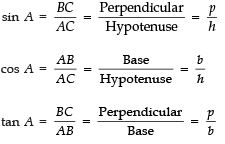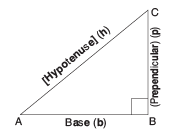Reciprocals of the above t-ratios are: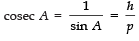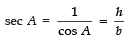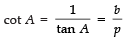NOTE:
I. ‘sin θ ’ is a single symbol and ‘sin’ cannot be detached from ‘θ ’. sin θ ≠ sin × θ. This remark is true for other t-ratios also.
II. The values of the trigonometric ratios of an angle depend only on the magnitude of the angle and not on the lengths of the sides of the triangle.
III. In a right angle, the hypotenuse is the longest side, therefore, the value of sin A or cos A is always less than 1 or at the most equal to 1.

• Trigonometric Ratios of Some Specific Angles
The specific angles are 0°, 30°, 45°, 60° and 90°. Trigonometric ratios of these angles are given in the following table: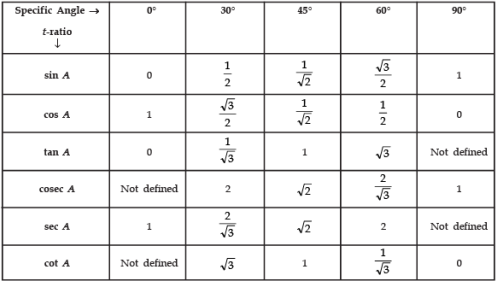NOTE:
I. The value of sin A increases from 0 to 1, as A increases from 0° to 90°.
II. The value of cos A decreases from 1 to 0, as A increases from 0° to 90°.
III. The value of tan A increases from 0 to ∞ , as A increases from 0° to 90°.
IV. √2 = 1.414 and √3 = 1.732.

• Trigonometric Identities
Since an equation is called an identity when it is true for all the values of the variables involved.
So, an equation involving trigonometric ratios of an angle is called a trigonometric identity, if it is true for all values of the angles involved. Some of the useful trigonometric identities:
(i) cos2 A + sin2 A = 1
(ii) 1 + tan2 A = sec2 A
(iii) cot2 A + 1 = cosec2 A
• Trigonometric Ratios of Complementary Angles
Two angles are said to be complementary if their sum equals 90°.
In a right angled Δ, if ∠B = 90°, then ∠A + ∠C = 90, i.e. ∠A and ∠C form a pair of complementary angles. Thus, ∠A and (90°– ∠A) are complementary angles.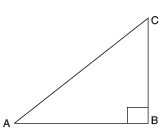Let us remember that:
(i) sin (90° − A) = cos A
(ii) cos (90° − A) = sin A
(iii) tan (90° − A) = cot A
(iv) cot (90° − A) = tan A
(v) sec (90° − A) = cosec A
(vi) cosec (90° − A) = sec A

NOTE:
I. tan 0° = cot 90° = 0
II. sec 0° = cosec 90° = 1
III. sec 90°, cosec 0°, cot 0° and tan 90° are not defined.

Offer running on EduRev: Apply code STAYHOME200 to get INR 200 off on our premium plan EduRev Infinity!

,

,

,

,

,

,

,

,

,

,

,

,

,

,

,

,

,

,

,

,

,

;